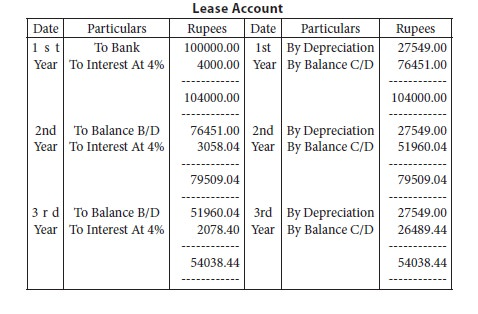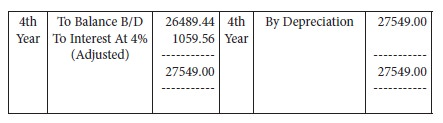Home | ARTS | Accounting For Managers | Annuity Method Of Depreciation and Summary

# Annuity Method Of Depreciation and Summary

Posted On :  26.01.2018 08:15 am

Under the first two methods of depreciation the interest aspect has been ignored. Under this method, the amount spent on the acquisition of an asset is regarded as investment which is assumed to earn interest at a certain rate.

Every year the asset is debited with the amount of interest and credited with the amount of depreciation. This interest is calculated on the debit balance of the asset account at the beginning of the year. The amount to be written off as depreciation is calculated from the annuity table an extract of which is given below:

 Years 3% 3.5% 4% 4.5% 5% 3 0.353530 0.359634 0.360349 0.363773 0.367209 4 0.269027 0.272251 0.275490 0.278744 0.282012 5 0.218355 0.221481 0.224627 0.227792 0.230975

The amount to be written off as depreciation is ascertained from

the annuity table and the same depends upon the rate of interest and the period over which the asset is to be written off. The rate of interest and the amount of depreciation would be adjusted in such a way that at the end of its working life, the value of the asset would be reduced to nil or its scrap value.

Evaluation:

This method has the merit of treating purchase of an asset as an investment within the business, and the same is supposed to earn interest. However, calculations become difficult when additions are made to the asset. The method is suitable only for long leases and other assets to which additions are not usually made and as such in case of machinery, this method is not found suitable.

Illustration 4:

A lease is purchased for a term of 4 years by payment of rs.1,00,000. It is proposed to depreciate the lease by annuity method charging 4% interest. If annuity of re.1 for 4 years at 4% is 0.275490, show the lease account for the full period.

Amount of annual depreciation =rs.1,00,000 x re.0.275490

= rs.27,549Summary

Though depreciation to a common man means a fall in the value of an asset, actually it is not a process of valuation. It is a process of cost allocation. Through depreciation accounting the cost of a tangible asset less salvage value, if any, is distributed over the estimated useful life of the asset. Depreciation is to be accounted to know the true profit earned by the concern, to exhibit a true and fair view of the state of assets of the concern and to provide funds for replacement of the asset when it is worn out. Among the number of methods of depreciation available three methods, viz. Straight line method, diminishing balance method and annuity method are discussed.

Tags : Accounting For Managers - Depreciation
Last 30 days 1390 views